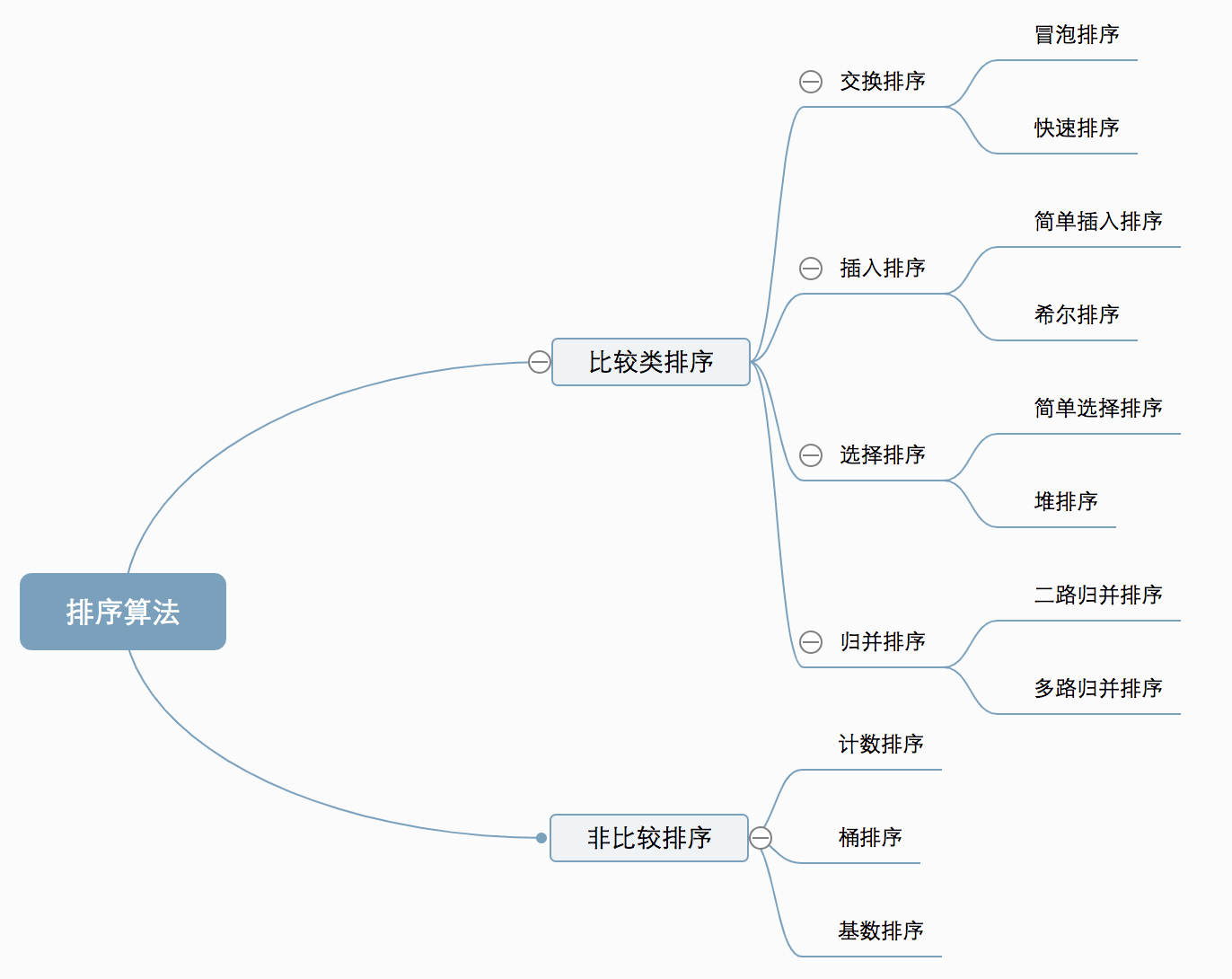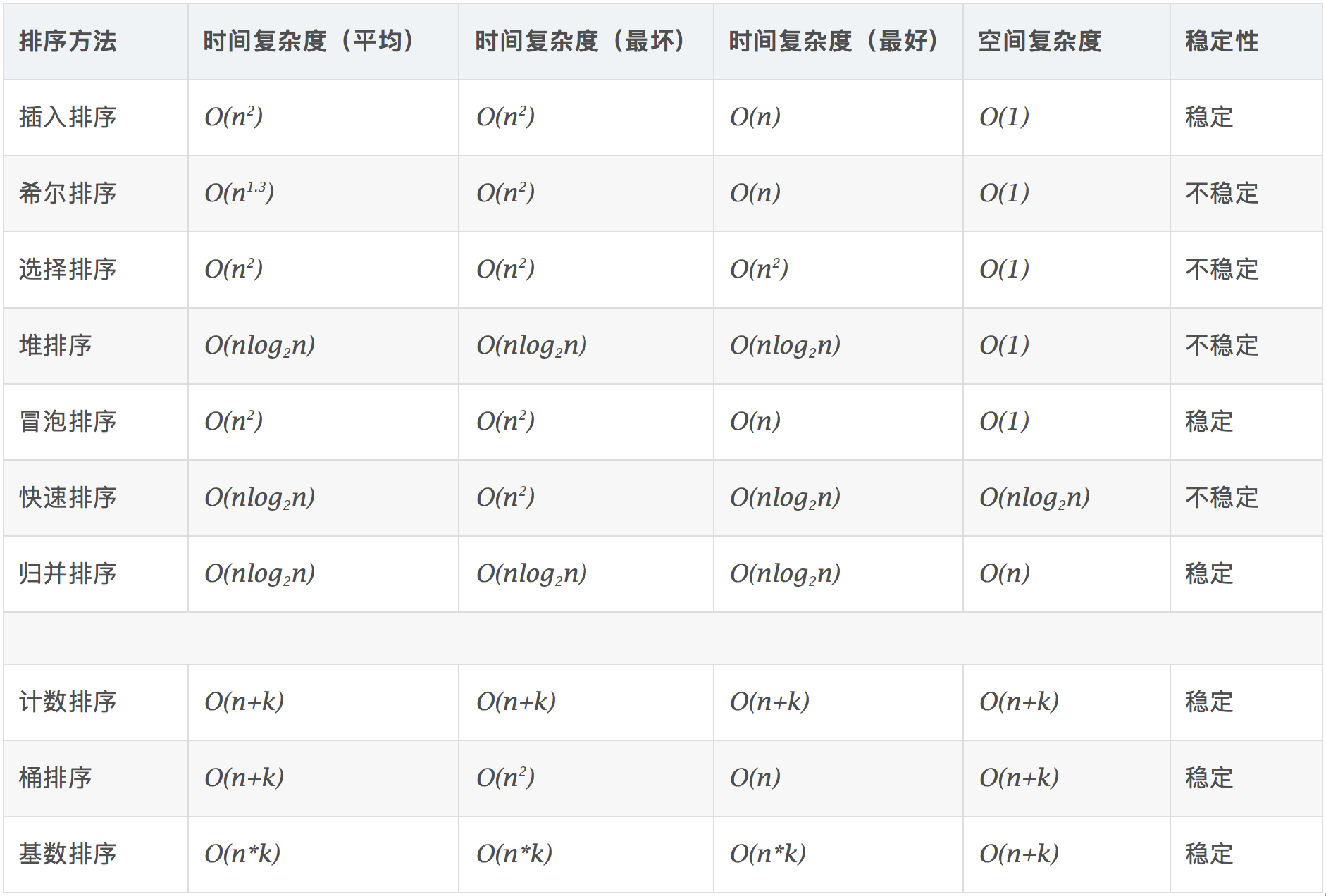# 排序算法总结

## Sorting Algorithms Summary

Posted by S.L on September 14, 2019

### 总结

#### 算法分类

• 比较类排序：通过比较来决定元素间的相对次序，由于其时间复杂度不能突破O(nlogn)，因此也称为非线性时间比较类排序。
• 非比较类排序：不通过比较来决定元素间的相对次序，它可以突破基于比较排序的时间下界，以线性时间运行，因此也称为线性时间非比较类排序。#### 复杂度#### 相关概念

• 稳定：如果a原本在b前面，而a=b，排序之后a仍然在b的前面。
• 不稳定：如果a原本在b的前面，而a=b，排序之后 a 可能会出现在 b 的后面。
• 时间复杂度：对排序数据的总的操作次数。反映当n变化时，操作次数呈现什么规律。
• 空间复杂度：是指算法在计算机内执行时所需存储空间的度量，它也是数据规模n的函数。

### 冒泡排序Bubble Sort

• 一共冒泡 `len - 1` 次，最后一次时待比较集合只有一个元素，已经是排好序不用再比较
• 内层每一轮进行比较的元素依次减少，冒泡了几次就减少几次， 这里用 `len - 1 - i` 来控制
• 交换不符合条件的相邻位置的元素，每一轮保证将最大或最小的元素「交换」到当前轮最后的位置
``````public static void bubbleSort(int arr[]){
int n = arr.length;
for (int i = 0; i < n - 1 ; i++) {
boolean isSort = true;
for (int j = 0; j < n - 1 - i ; j++) {
int temp = 0;
// 升序排列
if(arr[j] > arr[j + 1]){
temp = arr[j];
arr[j] = arr[j + 1];
arr[j + 1] = temp;
isSort = false;
}
}
// 如果遍历了一圈发现没有交换过数据，证明已经有序直接返回
if (isSort) {
break;
}
}
}
``````

### 选择排序Selection Sort

• 一共选择 `len - 1` 次， 最后一次时待比较集合只有一个元素，已经是排好序不用再比较
• 依次从第 `i` 下标位置的元素开始和后面的元素依次比较，找比它更小的元素，如果有，则交换数组中的位置
• 此时第 `i` 下标位置的数已经是当前待选择数据中最小的， `i` 递增，继续比较它后面的元素

``````public static void selectionSort(int arr[]) {
int n = arr.length;
for (int i = 0; i < n - 1; i++) {
// 本轮最小元素的下标
int min = i;
// 从后面依次对比找到最小的值的位置
for (int j = i + 1; j < n ; j++) {
if (arr[j] < arr[min]) {
min = j; // 找最小值
}
}
// 当前轮待更新的位置已经是所有后续中最小的，无需交换
if (min != i) {
// 交换位置
int temp = arr[i];
arr[i] = arr[min];
arr[min] = temp;
}
}
}
``````

• 选择排序的每一轮最多只交换一次，而冒泡排序每一轮可能要交换`len-1-i`次，即第一个位置需要和后面所有位置都交换一遍

• 选择排序的每一轮需要比较完所有的未排序元素，而冒泡排序在上一轮全部比较完毕后如果未发生交换，则无需开始下轮的比较

### 插入排序Insertion Sort

``````public static void insertionSort(int[] arr) {
int n = arr.length;
// 直接从第二位置的数据开始与其前面的集合倒序比较，所谓的插入是插入一个已经存在的集合（最小长度为1）中
for (int i = 1; i < n; ++i) {
// 保存当前待插入集合中的数据
int value = arr[i];
// 保存单签待插入数据的前一个位置
int j = i - 1;
// 倒序与待插入位置元素之前的元素进行比较，找到最适合value的位置
for (; j >= 0; j--) {
// 如果小于则腾出该位置
if (arr[j] > value) {
// 将j位置的数据向后一位赋值，给i位置数据留出位置
arr[j + 1] = arr[j];
} else {
// 退出，前面的已经是排好序的了
break;
}
}
// 插入本轮待比较数据到最合适的位置，如果该位置未改变，则无需移动
if (j + 1 != i) {
// 此时j+1和j+2的值是一样的，因为j+1位置的元素刚移动到了j+2位置
arr[j + 1] = value;
}
}
}
``````

• 前者每轮的比较次数是`依次增大`的，并且比较是`从后往前`依次比较，每一轮的结果保证此轮所有待排序元素是排好序的
• 后者两个每轮的比较次数是`依次减少`的，并且比较是`从前往后`依次比较，每一轮的结果保证此轮所有待排序元素范围「之后的」数据是排好序的， 如冒泡排序是「尾部」是已经排好序的，只需要调整「头部」，而插入排序是「头部」是已经排好序的，需要通过尾部的新元素继续调整「头部」。

### 希尔排序Shell’s Sort

``````
public class ShellSort {

/**
* 希尔排序 针对有序序列在插入时采用交换法
* @param arr
*/
public static void sortBySwap(int []arr){
// 增量gap，并逐步缩小增量
for(int gap = arr.length / 2; gap > 0; gap /= 2){
// 从第gap个元素开始，逐个对每个元素以及其所在组中的数据进行直接插入排序操作
for(int i = gap; i < arr.length; i++){
int j = i;
// 依次从后向前做比较，把后面的小元素移动到前面的位置
// 不满足时直接退出，说明前面的元素已经排好序了
while(j - gap >= 0 && arr[j] < arr[j - gap]){
// 插入排序采用交换法
swap(arr, j, j - gap);
j -= gap;
}
}
}
}

public static void swap(int []arr, int a, int b){
arr[a] = arr[a]+arr[b];
arr[b] = arr[a]-arr[b];
arr[a] = arr[a]-arr[b];
}

/**
* 希尔排序 针对有序序列在插入时采用移动法。
* @param arr
*/
public static void sortByMove(int []arr){
// 增量gap，并逐步缩小增量
for(int gap = arr.length/2; gap > 0; gap /= 2){
// 从第gap个元素开始，逐个对每个元素以及其所在组中的数据进行直接插入排序操作
for(int i = gap; i < arr.length; i++){
int j = i;
int temp = arr[j];
if(arr[j] < arr[j - gap]){
while(j - gap >= 0 && temp < arr[j - gap]){
//移动法，减少交换法中的赋值操作
arr[j] = arr[j - gap];
j -= gap;
}
arr[j] = temp;
}
}
}
}

}
``````

### 快速排序Quick Sort

• 跳出条件是 `endIndex <= startIndex`，此时已经无需再比较（和移动），因为待排序集合只有一个元素了
• 执行逻辑：在当前数据范围内找到一个 `pivot` ，以该点为中心， `pivot` 怎么选呢？比如以第一个位置的元素当做基准数，然后分别用两个指针在最左侧和最右侧依次向中间的方向寻找第一个大于或小于 `pivot` 的数的下标。 如果找到则将两个位置的数据进行交换，保证排序的规则，然后继续让两个指针按照原来的方向继续寻找，一直到两个指针相遇， 则相遇位置即为当前轮次的pivot位置元素需要放到的位置，此时其他所有位置都满足pivot左侧小于它右侧大于它，所以全局层面pivot位置的元素的位置已经确定了。 剩下的左右两侧的元素集合继续按照这个思路继续处理即可。
``````// 递归处理，因为每一次处理的元素的范围不同，则最好定义一个新的方法增加下标参数
// 但注意arr的引用一直都没有变，即内存地址一直没有变，变的是内存地址内数组元素位置和值
public static void quickSort(int[] arr) {
quickSort0(arr, 0, arr.length - 1);
}

private static void quickSort0(int[] arr, int startIndex, int endIndex) {
if (endIndex <= startIndex) {
return;
}
// 切分点计算
int pivotIndex = partition(arr, startIndex, endIndex);
// 分治divide
quickSort0(arr, startIndex, pivotIndex - 1);
quickSort0(arr, pivotIndex + 1, endIndex);
}

private static int partition(int[] arr, int low, int high) {
int left = low;
int right = high;
int pivot = arr[low]; // 取第一个元素为基准值

while (true) {
// 从左往右扫描，找到比pivot大的则退出
while (arr[left] <= pivot) {
left++;
if (left == right) {
break;
}
}

// 从右往左扫描，找到比pivot小于等于的则退出
while (pivot < arr[right]) {
right--;
if (left == right) {
break;
}
}

// 左右指针相遇
if (left >= right) {
break;
}

// 交换左右指针下标对应的数据，使得满足左小于右大于pivot
int temp = arr[left];
arr[left] = arr[right];
arr[right] = temp;
}

// 将基准值插入序列
int temp = arr[low];
arr[low] = arr[right];
arr[right] = temp;
return right;
}
``````

``````void QuickSort(int *arr, int low, int high)
{
if (low < high)
{
int i = low;
int j = high;
/*默认使用最低位的元素作为比较值*/
int k = arr[low];
while (i < j)
{
/*从右向左找第一个小于k的数*/
while(i < j && arr[j] >= k)
{
j--;
}

if(i < j)
{
arr[i++] = arr[j];
}

/*从左向右找第一个大于等于k的数*/
while(i < j && arr[i] < k)
{
i++;
}

if(i < j)
{
arr[j--] = arr[i];
}
}

/*将pivot值赋值到i位置，此时i==j*/
arr[i] = k;

/*递归调用*/
/*排序k左边*/
QuickSort(arr, low, i - 1);
/*排序k右边*/
QuickSort(arr, i + 1, high);
}
}
``````

C语言和Java语言的实现在交换i和j位置数据的时候有一点点不一样，C是按照一个方向判断，如果不满足则直接保存到另外一侧，但保留当前位置等待另外一侧不满足的赋值过来。 Java版本的是直接找到两侧不满足条件的位置后，直接进行i和j位置的交换，本质上和C算法是一样的。

#### 权衡

• 快速排序算法中，序列被划分为越来越短的片段。若序列已经很短，例如短于几个元素，快排还需要做几次递归调用（进栈、出栈）。 这些赋值操作很耗时，表现在复杂度描述中忽略了的常量因子。对于很短的序列，采用插入排序，效果很可能优于快速排序。
• 归并排序正好与快排相反，是从短的有序序列归并出越来越长的序列。从很多个各自包含一个元素的序列出发，通过几遍归并得到最终的有序序列， 这其中需要做许多函数调用工作。与这几个元素做简单插入排序相比，归并排序消耗时间会更多。

### 堆排序Heap Sort

• 满二叉树：除了叶子节点，所有的节点的左右孩子都不为空，就是一棵满二叉树
• 完全二叉树：不一定是一个满二叉树，但它不满的那部分一定在右下侧

• 必须是完全二叉树
• 任一结点的值是其子树所有结点的最大值最小值

• 步骤一

• 步骤二

``````public static void heapSort(int[] arr) {
int totalLength = arr.length;
// 构建堆
buildHeap(arr, totalLength);
int newLength = totalLength;
for ( int i = totalLength - 1; i > 0; i-- ) {
// 将堆顶元素（当前堆中最大）与当前堆末尾元素调换
int temp = arr;
arr = arr[i];
arr[i] = temp;
// 新堆数组长度-1 隐藏全局数组尾部已全局有序元素
newLength--;
// 新的堆不满足条件，需要将「堆顶元素」下沉，将该节点下的最大值调整到堆顶上来
sink(arr, 0, newLength); // length用于控制每一轮调整堆结构时排除的部分的边界，即已有序部分不参与堆的重建
}
}

// 对已有数组arr或者说是「无序堆」进行构建大顶堆结构
private static void buildHeap(int[] arr, int length) {
// 从第一个非叶子节点开始调整，即length/2 - 1的位置开始调整子堆，调整完毕后，继续调整每个小于i的位置的子堆结构
// 注意因为堆是完全二叉树，所以一定满足i--后的位置一定是非叶子节点
// Q:为什么第一次建堆需要从第一个非叶子节点开始依次调整每一个子堆，而后续交换堆顶元素后可以直接从堆顶位置开始调整？
// A:因为之前的子堆已经建立好了堆结构，只需从第一个子节点开始调整
for (int i = length / 2 - 1; i >= 0; i--) {
sink(arr, i, length);
}
}

// 下沉调整，如果调整后下一层仍不满足条件则继续调整，一直递归处理
private static void sink(int[] arr, int index, int length) {
int leftChild = 2 * index + 1; // 左子节点下标
int rightChild = 2 * index + 2; // 右子节点下标
int sinkMe = index; //要调整的节点下标

// 下沉到左边
if (leftChild < length && arr[leftChild] > arr[sinkMe]) {
sinkMe = leftChild;
}

// 如果右边更大，则改为下沉到右边，即让左右孩子中最大的往上浮
if (rightChild < length && arr[rightChild] > arr[sinkMe]) {
sinkMe = rightChild;
}

// 如果下标不相等了，证明index需要和sinkMe位置的孩子进行交换
if (sinkMe != index) {
// 交换值
int temp = arr[index];
arr[index] = arr[sinkMe];
arr[sinkMe] = temp;

// sinkMe此时已经下沉，从该节点开始继续处理下沉逻辑，递归
sink(arr, sinkMe, length); // 递归
}
// 如果相同，则证明已经满足节点是左右子树中最大的节点值，退出
}

``````

a.将无序序列构建成一个堆，根据是得到升序or降序结果集的需求选择使用大顶堆或小顶堆;

b.将堆顶元素与末尾元素交换，将最大元素”沉”到数组末端;

c.重新调整（不包括移除的元素集合）结构，使其满足堆定义，然后继续交换堆顶元素与当前轮末尾元素，反复执行调整+交换步骤，直到整个序列有序。

#### 复杂度

• 大约一半的结点深度为 `d-1` ，不移动(叶)
• 四分之一的结点深度为 `d-2` ，而它们至多能向下移动一层
• 树中每向上一层，结点的数目为前一层的一半，而子树高度加一

``````public class MinHeap <E extends Comparable<E>> {
private ArrayList<E> data;

public MinHeap(int capacity) {
data = new ArrayList<>(capacity);
}

public MinHeap() {
data = new ArrayList<>();
}

// 返回堆中的元素个数
public int size() {
return data.size();
}

// 返回一个布尔值, 表示堆中是否为空
public boolean isEmpty() {
return data.isEmpty();
}

// 返回完全二叉树的数组表示中，一个索引所表示的元素的父亲节点的索引
public int parent(int i) {
return (i -1) / 2;
}

// 返回完全二叉树的数组表示中，一个索引所表示的元素的左孩子节点的索引
public int leftChild(int i) {
return i * 2 + 1;
}

// 返回完全二叉树的数组表示中，一个索引所表示的元素的右孩子节点的索引
public int rightChild(int i) {
return i * 2 + 2;
}

public void swap(int i, int j) {
if (i < 0 || i >= size() || j < 0 || j >= size())
throw new IllegalArgumentException("Index is illegal.");

E temp = data.get(i);
data.set(i, data.get(j));
data.set(j, temp);
}

// 特性1：新插入的元素首先放在数组最后，保持完全二叉树的特性
siftUp(data.size() - 1);
}

private void siftUp(int i) {
// 特性2：比较插入值和其父结点的大小关系，小于父结点则用父结点替换当前值，index位置上升为父结点
// 当上浮元素大于父亲，继续上浮。并且不能上浮到0之上
// 直到i 等于 0 或 比 父亲节点小了。
while (i > 0 && data.get(i).compareTo(data.get(parent(i))) > 0) {
// 数组Array中添加方法swap
this.swap(i, parent(i));
i = parent(i); // 这句话让i来到新的位置，使得循环可以查看新的位置是否还要大。
}
}

public E findMin() {
return data.get(0);
}

public E extratMin() {
E ret = findMin();
swap(0, size() - 1); // 0位置元素和最后一个元素互换。
data.remove(size() - 1); // 删除此时的最后一个元素(最小值)
siftDown(0); // 对于0处进行siftDown操作
return ret;
}

// k位置元素下移
private void siftDown(int k) {
while (leftChild(k) < size()) {
int j = leftChild(k); // 在此轮循环中,data[k]和data[j]交换位置
if (j + 1 < size() && data.get(j + 1).compareTo(data.get(j)) < 0) {
j ++;
// data[j] 是 leftChild 和 rightChild 中的最小值
// 该值将和data[k]进行比较，保证每次交换都是交换当前最小的子节点
}
if (data.get(j).compareTo(data.get(k)) >= 0) {
// 如果parent已经小于最小值，则直接退出
break;
}
// 否则交换k和j的数据
swap(k, j);
// 让k从j的位置继续和子节点去比较
k = j;
}
}
}
``````

### 归并排序Merge Sort

Recursively decompose problem until it is small enough for sequential

• 1、判断参数的有效性，也就是递归的出口，即当只有一个元素时；
• 2、否则，直接把当前数组平分成两个子数组；
• 3、递归调用本函数，最后划分到数组中只有一个元素，这也意味着数组是有序的了；
• 4、然后调用排序函数，把两个有序的数组合并成一个有序的数组；
• 5、排序函数的步骤，让两个数组的元素进行比较，把大的/小的元素存放到临时数组中，如果有一个数组的元素被取光了， 那就直接把另一数组的元素放到临时数组中，然后把临时数组中的元素都复制到实际的数组中；
``````public static void mergeSort(int[] arr) {
int[] tempArr = new int[arr.length]; // 初始化临时变量，全局共享
mergeSort0(arr,  tempArr,  0,  arr.length-1);
}

/**
* 归并排序，将arr数组中从startIndex到endIndex部分的数据进行排序
* @param arr 排序数组
* @param tempArr 临时存储数组
* @param startIndex 排序起始位置
* @param endIndex 排序终止位置
*/
private static void mergeSort0(int[] arr, int[] tempArr, int startIndex, int endIndex){
// 退出条件，左右指针相逢，待合并数组只有一个元素
if(endIndex <= startIndex){
return;
}
// 二路归并，计算中部下标
int middleIndex = startIndex + (endIndex - startIndex) / 2; // 注意防止溢出

// divide
// 分解为左右两部分并各自进行递归排序
mergeSort0(arr, tempArr, startIndex, middleIndex);
mergeSort0(arr, tempArr, middleIndex + 1, endIndex);

// conquer
// 注意merge前[startIndex, middleIndex]和[middleIndex+1, endIndex]两部分已经分别由下一层merge()递归排好序了
merge(arr, tempArr, startIndex, middleIndex, endIndex);
}

/**
* 归并，将左右两部分已经排好序的数据依次进行比较后重新赋值给arr中
* e.g. 0235 4678 -> 02345678
* @param arr 待排序数组
* @param tempArr 临时存储数组，用于赋值排序
* @param startIndex 归并起始位置
* @param middleIndex 归并中间位置
* @param endIndex 归并终止位置
*/
private static void merge(int[] arr,  int[] tempArr,  int startIndex,  int middleIndex,  int endIndex) {
// 复制本次start~end范围内的数据到临时数组
// 只将待合并区间的数据复制到临时数组即可，其他相邻同长度的区域由上一层merge来处理合并
for (int s = startIndex; s <= endIndex; s++) {
tempArr[s] = arr[s];
}

int l = startIndex;
int r = middleIndex + 1;

// 选择排序，局部有序
for (int k = startIndex; k <= endIndex; k++) {
if(l > middleIndex){ // 左头指针超过了自己的边界
arr[k] = tempArr[r++];
} else if (r > endIndex){ // 右头指针超过了自己的边界
arr[k] = tempArr[l++];
} else if (tempArr[r] < tempArr[l]){ // 两部分比较取最小
arr[k] = tempArr[r++];
} else {
arr[k] = tempArr[l++];
}
}
}
``````

#### 复杂度分析

• 时间复杂度 `merge` 方法中只有一个 `for` 循环，直接就可以得出每次合并的时间复杂度为 `O(n)` ，而分解数组每次「对半切割」， 属于对数时间 `O(log n)` ，总的时间复杂度为 `O(nlogn)`

• 空间复杂度 如果对`merge`的每个递归调用都声明一个临时数组，那么任一时刻可能会有`logn`个临时数组处于活动期，这对小内存机器是致命的。 另一方面，如果`merge`动态分配并释放最小量临时空间，会增加时间开销。 因此在任一时刻只需要一个临时数组活动，可以使用该临时数组的任意部分（通过下标控制）在不同的分割阶段；我们将使用和输入数组`arr`相同的部分。 这样的话，该算法的空间占用为`o(n)`，即待排序的数组元素个数。

#### 归并和快排

• 快速排序：先把数组粗略的排序成两个子数组（只有```pivot ```位置的数据是排好序的并且它大于左侧所有数据，小于右侧所有数据），然后递归再将pivot 左右两侧的数组以相同的方式继续寻找对应的pivot和排序好左右两侧的数据，直到左右子数组里面只有一个元素，那么就自然整个集合就排好序了， 可以总结为：先(基于pivot粗略)排序再递归(粗略排序后的pivot的左半部分和右半部分，两边彼此独立)，每一轮给pivot元素寻找存放位置的时候 都会随时做数据的移动，所以是边divide边conquer；
• 归并排序：先什么都不管，把数组分为两个子数组，一直递归把数组划分为两个子数组，直到数组里只有一个元素，这时候才开始排序， 让两个数组间排好序，依次按照递归的返回来把两个数组进行排好序，到最后就可以把整个数组排好序，可以总结为：先递归再排序，divide and conquer比较完美的提现；

• https://cxyxiaowu.com/articles/2019/06/11/1560233679033.html
• https://www.cnblogs.com/chengxiao/p/6129630.html
• https://ipine.me/2018-11-04/
• https://blog.csdn.net/u013074465/article/details/42043967
• https://www.cnblogs.com/chengxiao/p/6104371.html